# Train SAC Agent for Ball Balance Control

This example shows how to train a soft actor-critic (SAC) reinforcement learning agent to control a robot arm for a ball-balancing task.

### Introduction

The robot arm in this example is a Kinova Gen3 robot, which is a seven degree-of-freedom (DOF) manipulator. The arm is tasked to balance a ping pong ball at the center of a flat surface (plate) attached to the robot gripper. Only the final two joints are actuated and contribute to motion in the pitch and roll axes as shown in the following figure. The remaining joints are fixed and do not contribute to motion.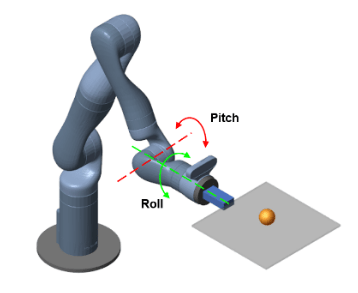Open the Simulink® model to view the system. The model contains a Kinova Ball Balance subsystem connected to an RL Agent block. The agent applies an action to the robot subsystem and receives the resulting observation, reward, and is-done signals.

`open_system("rlKinovaBallBalance")`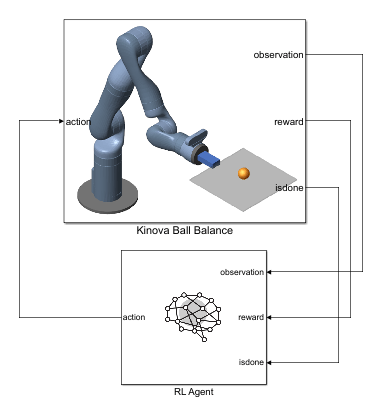View to the Kinova Ball Balance subsystem.

`open_system("rlKinovaBallBalance/Kinova Ball Balance")`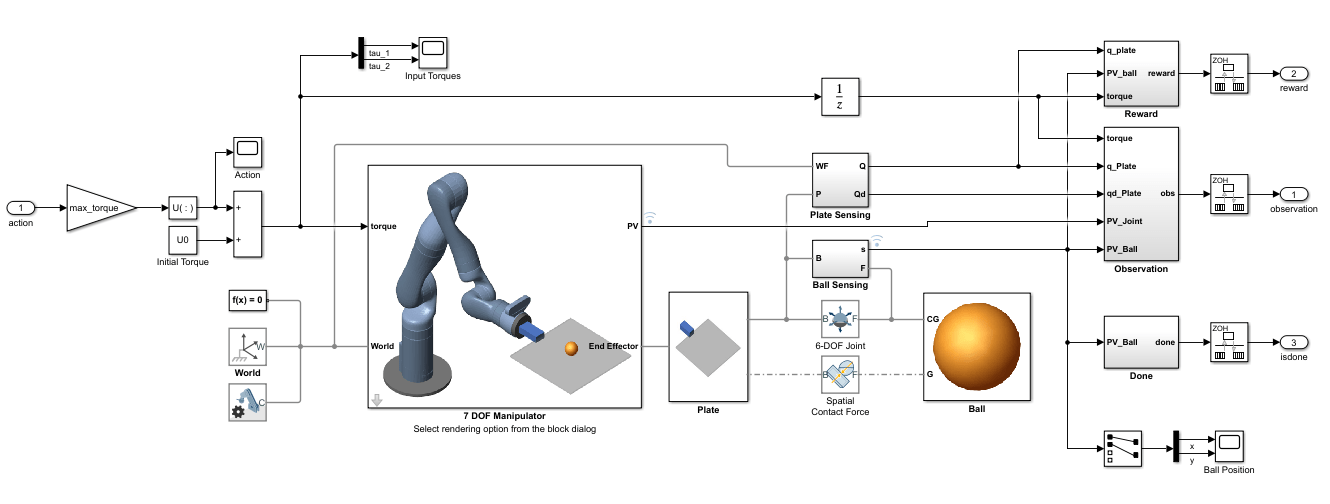In this model:

• The physical components of the system (manipulator, ball, and plate) are modeled using Simscape™ Multibody™ components.

• The plate is constrained to the end effector of the manipulator.

• The ball has six degrees of freedom and can move freely in space.

• Contact forces between the ball and plate are modeled using the Spatial Contact Force block.

• Control inputs to the manipulator are the torque signals for the actuated joints.

If you have the Robotics System Toolbox Robot Library Data support package, you can view a 3-D animation of the manipulator in the Mechanics Explorer. To do so, open the 7 DOF Manipulator subsystem and set its Visualization parameter to `3D Mesh`. If you do have the support package installed, set the Visualization parameter to `None`. To download and install the support package, use the Add-On Explorer. For more information see Get and Manage Add-Ons.

Create the parameters for the example by running the `kinova_params` script included with this example. When you have the Robotics System Toolbox Robot Library Data support package installed, this script also adds the necessary mesh files to the MATLAB® path.

`kinova_params`

### Define Environment

To train a reinforcement learning agent, you must define the environment with which it will interact. For the ball balancing environment:

• The observations are represented by a 22 element vector that contains information about the positions (sine and cosine of joint angles) and velocities (joint angle derivatives) of the two actuated joints, positions (x and y distances from plate center) and velocities (x and y derivatives) of the ball, orientation (quaternions) and velocities (quaternion derivatives) of the plate, joint torques from the last time step, ball radius, and mass.

• The actions are normalized joint torque values.

• The sample time is ${\mathit{T}}_{\mathit{s}}=0.01\mathit{s}$, and the simulation time is ${\mathit{T}}_{\mathit{f}}=10\mathit{s}$.

• The simulation terminates when the ball falls off the plate.

• The reward ${\mathit{r}}_{\mathit{t}}$ at time step $\mathit{t}$ is given by:

`$\begin{array}{l}{\mathit{r}}_{\mathit{t}}={\mathit{r}}_{\mathrm{ball}}+{\mathit{r}}_{\mathrm{plate}}+{\mathit{r}}_{\mathrm{action}}\\ {\mathit{r}}_{\mathrm{ball}}={\mathit{e}}^{-0.001\left({\mathit{x}}^{2}+{\mathit{y}}^{2}\right)}\\ {\mathit{r}}_{\mathrm{plate}}=-0.1\left({\varphi }^{2}+{\theta }^{2}+{\psi }^{2}\right)\\ {\mathit{r}}_{\mathrm{action}}=-0.05\left({\tau }_{1}^{2}+{\tau }_{2}^{2}\right)\end{array}$`

Here, ${\mathit{r}}_{\mathrm{ball}}$ is a reward for the ball moving closer to the center of the plate, ${\mathit{r}}_{\mathrm{plate}}$ is a penalty for plate orientation, and ${\mathit{r}}_{\mathrm{action}}$ is a penalty for control effort. $\varphi$, $\theta$, and $\psi$ are the respective roll, pitch, and yaw angles of the plate in radians. ${\tau }_{1}$ and ${\tau }_{2}$ are the joint torques.

Create the observation and action specifications for the environment using continuous observation and action spaces.

```numObs = 22; % Number of observations numAct = 2; % Number of actions obsInfo = rlNumericSpec([numObs 1]); actInfo = rlNumericSpec([numAct 1]); actInfo.LowerLimit = -1; actInfo.UpperLimit = 1;```

Create the Simulink environment interface using the observation and action specifications. For more information on creating Simulink environments, see `rlSimulinkEnv`.

```mdl = "rlKinovaBallBalance"; blk = mdl + "/RL Agent"; env = rlSimulinkEnv(mdl,blk,obsInfo,actInfo);```

Specify a reset function for the environment using the `ResetFcn` parameter.

`env.ResetFcn = @kinovaResetFcn;`

This reset function (provided at the end of this example) randomly initializes the initial x and y positions of the ball with respect to the center of the plate. For more robust training, you can also randomize other parameters inside the reset function, such as the mass and radius of the ball.

Specify the sample time `Ts` and simulation time `Tf`.

```Ts = 0.01; Tf = 10;```

### Create Agent

The agent in this example is a soft actor-critic (SAC) agent. SAC agents have critics that approximate the expectation of the value function given the states and actions and an actor that models a stochastic policy. The agent selects an action based on this policy. For more information on SAC agents, see Soft Actor-Critic Agents.

The SAC agent in this example uses two critics to learn the optimal Q-value function. Using two critics helps avoid overfitting when learning the Q-function. To create the critics, first create a deep neural network with two inputs (the observation and action) and one output. For more information on creating deep neural networks for reinforcement learning agents, see Create Policies and Value Functions.

```% Set the random seed for reproducibility. rng(0) % Define the network layers. cnet = [ featureInputLayer(numObs,"Normalization","none","Name","observation") fullyConnectedLayer(128,"Name","fc1") concatenationLayer(1,2,"Name","concat") reluLayer("Name","relu1") fullyConnectedLayer(64,"Name","fc3") reluLayer("Name","relu2") fullyConnectedLayer(32,"Name","fc4") reluLayer("Name","relu3") fullyConnectedLayer(1,"Name","CriticOutput")]; actionPath = [ featureInputLayer(numAct,"Normalization","none","Name","action") fullyConnectedLayer(128,"Name","fc2")]; % Connect the layers. criticNetwork = layerGraph(cnet); criticNetwork = addLayers(criticNetwork, actionPath); criticNetwork = connectLayers(criticNetwork,"fc2","concat/in2");```

View the critic neural network.

`plot(criticNetwork)`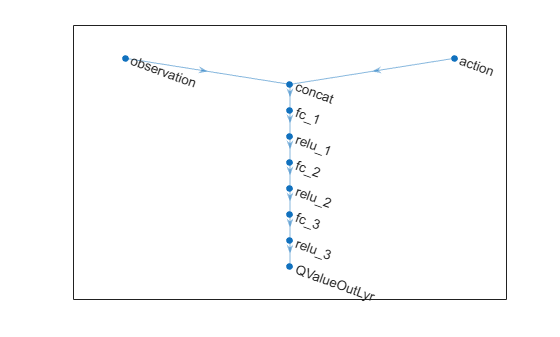When using two critics, a SAC agent requires them to have different initial parameters. Create and initialize two `dlnetwork` objects.

```criticdlnet = dlnetwork(criticNetwork,'Initialize',false); criticdlnet1 = initialize(criticdlnet); criticdlnet2 = initialize(criticdlnet);```

Create the critic functions using `rlQValueFunction`.

```critic1 = rlQValueFunction(criticdlnet1,obsInfo,actInfo, ... "ObservationInputNames","observation"); critic2 = rlQValueFunction(criticdlnet2,obsInfo,actInfo, ... "ObservationInputNames","observation");```

The actor function in a SAC agent is stochastic actor with a continuous action space, which you define as an `rlContinuousGaussianActor` object. Create a deep neural network to model the actor policy.

```% Create the actor network layers. anet = [ featureInputLayer(numObs,"Normalization","none","Name","observation") fullyConnectedLayer(128,"Name","fc1") reluLayer("Name","relu1") fullyConnectedLayer(64,"Name","fc2") reluLayer("Name","relu2")]; meanPath = [ fullyConnectedLayer(32,"Name","meanFC") reluLayer("Name","relu3") fullyConnectedLayer(numAct,"Name","mean")]; stdPath = [ fullyConnectedLayer(numAct,"Name","stdFC") reluLayer("Name","relu4") softplusLayer("Name","std")]; % Connect the layers. actorNetwork = layerGraph(anet); actorNetwork = addLayers(actorNetwork,meanPath); actorNetwork = addLayers(actorNetwork,stdPath); actorNetwork = connectLayers(actorNetwork,"relu2","meanFC/in"); actorNetwork = connectLayers(actorNetwork,"relu2","stdFC/in");```

View the actor neural network.

`plot(actorNetwork)`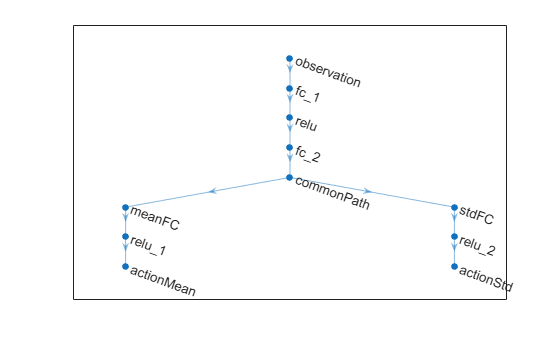Create the actor function using `rlContinuousGaussianActor`.

```actordlnet = dlnetwork(actorNetwork); actor = rlContinuousGaussianActor(actordlnet, obsInfo, actInfo, ... "ObservationInputNames","observation", ... "ActionMeanOutputNames","mean", ... "ActionStandardDeviationOutputNames","std");```

The SAC agent in this example trains from an experience buffer of maximum capacity 1e6 by randomly selecting mini-batches of size 128. The discount factor of 0.99 is close to 1 and therefore favors long term reward with respect to a smaller value. For a full list of SAC hyperparameters and their descriptions, see `rlSACAgentOptions`.

Specify the agent hyperparameters for training.

```agentOpts = rlSACAgentOptions( ... "SampleTime",Ts, ... "TargetSmoothFactor",1e-3, ... "ExperienceBufferLength",1e6, ... "MiniBatchSize",128, ... "NumWarmStartSteps",1000, ... "DiscountFactor",0.99);```

For this example the actor and critic neural networks are updated using the Adam algorithm with a learn rate of 1e-4 and gradient threshold of 1. Specify the optimizer parameters.

```agentOpts.ActorOptimizerOptions.Algorithm = "adam"; agentOpts.ActorOptimizerOptions.LearnRate = 1e-4; agentOpts.ActorOptimizerOptions.GradientThreshold = 1; for ct = 1:2 agentOpts.CriticOptimizerOptions(ct).Algorithm = "adam"; agentOpts.CriticOptimizerOptions(ct).LearnRate = 1e-4; agentOpts.CriticOptimizerOptions(ct).GradientThreshold = 1; end```

Create the SAC agent.

`agent = rlSACAgent(actor,[critic1,critic2],agentOpts);`

### Train Agent

To train the agent, first specify the training options using `rlTrainingOptions`. For this example, use the following options:

• Run each training for at most 5000 episodes, with each episode lasting at most `floor(Tf/Ts)` time steps.

• Stop training when the agent receives an average cumulative reward greater than 675 over 100 consecutive episodes.

• To speed up training set the `UseParallel` option to `true`. Doing so is optional and requires Parallel Computing Toolbox™ software.

```trainOpts = rlTrainingOptions(... "MaxEpisodes", 5000, ... "MaxStepsPerEpisode", floor(Tf/Ts), ... "ScoreAveragingWindowLength", 100, ... "Plots", "training-progress", ... "StopTrainingCriteria", "AverageReward", ... "StopTrainingValue", 675, ... "UseParallel", false);```

For parallel training, specify a list of supporting files. These files are required to model the Kinova robot in the parallel workers when the CAD geometry rendering option is selected.

```if trainOpts.UseParallel trainOpts.ParallelizationOptions.AttachedFiles = [pwd,filesep] + ... ["bracelet_with_vision_link.STL"; "half_arm_2_link.STL"; "end_effector_link.STL"; "shoulder_link.STL"; "base_link.STL"; "forearm_link.STL"; "spherical_wrist_1_link.STL"; "bracelet_no_vision_link.STL"; "half_arm_1_link.STL"; "spherical_wrist_2_link.STL"]; end```

Train the agent using the `train` function. Training this agent is a computationally intensive process that takes several minutes to complete. To save time while running this example, load a pretrained agent by setting `doTraining` to `false`. To train the agent yourself, set `doTraining` to `true`.

```doTraining = false; if doTraining stats = train(agent,env,trainOpts); else load("kinovaBallBalanceAgent.mat") end```

A snapshot of training progress is shown in the following figure. You can expect different results due to randomness in the training process.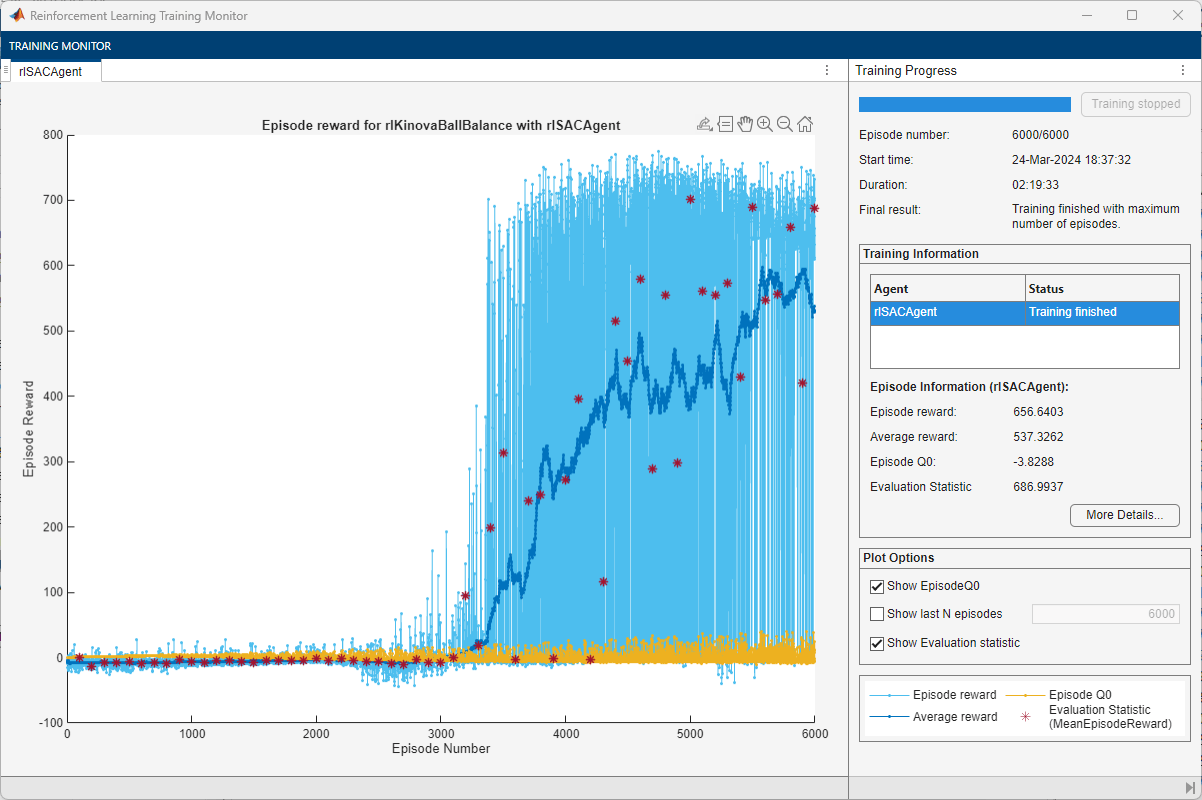### Simulate Trained Agent

To validate the trained agent, run the Simulink model.

Define an arbitrary initial position for the ball with respect to the plate center. To view the agent performance in different situations, change this to other locations on the plate.

```ball.x0 = 0.10; ball.y0 = -0.10;```

Create a `Simulink.SimulationInput` object and set the initial ball position.

```in = Simulink.SimulationInput(mdl); in = setVariable(in,"ball",ball);```

Optionally, you can attach an animation function.

`in = setPostSimFcn(in,@animatedPath);`

Simulate the model.

`out = sim(in);`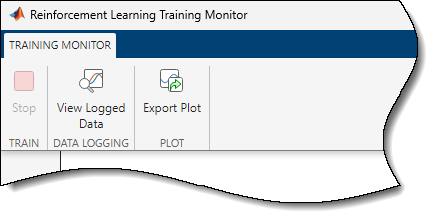View the trajectory of the ball using the Ball Position scope block.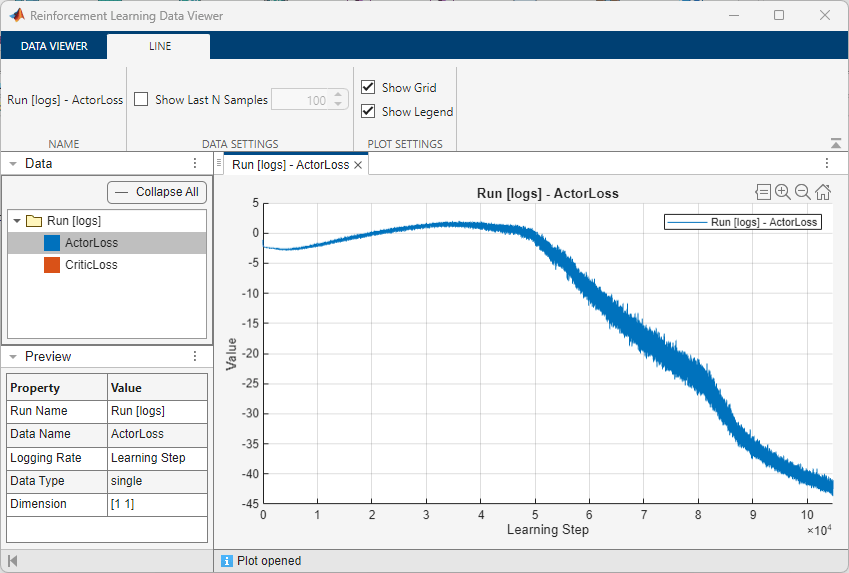### Environment Reset Function

```function in = kinovaResetFcn(in) % Ball parameters ball.radius = 0.02; % m ball.mass = 0.0027; % kg ball.shell = 0.0002; % m % Calculate ball moment of inertia. ball.moi = calcMOI(ball.radius,ball.shell,ball.mass); % Initial conditions. +z is vertically upward. % Randomize the x and y distances within the plate. ball.x0 = -0.125 + 0.25*rand; % m, initial x distance from plate center ball.y0 = -0.125 + 0.25*rand; % m, initial y distance from plate center ball.z0 = ball.radius; % m, initial z height from plate surface ball.dx0 = 0; % m/s, ball initial x velocity ball.dy0 = 0; % m/s, ball initial y velocity ball.dz0 = 0; % m/s, ball initial z velocity % Contact friction parameters ball.staticfriction = 0.5; ball.dynamicfriction = 0.3; ball.criticalvelocity = 1e-3; % Convert coefficient of restitution to spring-damper parameters. coeff_restitution = 0.89; [k, c, w] = cor2SpringDamperParams(coeff_restitution,ball.mass); ball.stiffness = k; ball.damping = c; ball.transitionwidth = w; in = setVariable(in,"ball",ball); % Randomize joint angles within a range of +/- 5 deg from the % starting positions of the joints. R6_q0 = deg2rad(-65) + deg2rad(-5+10*rand); R7_q0 = deg2rad(-90) + deg2rad(-5+10*rand); in = setVariable(in,"R6_q0",R6_q0); in = setVariable(in,"R7_q0",R7_q0); % Compute approximate initial joint torques that hold the ball, % plate and arm at their initial congifuration g = 9.80665; wrist_torque_0 = ... (-1.882 + ball.x0 * ball.mass * g) * cos(deg2rad(-65) - R6_q0); hand_torque_0 = ... (0.0002349 - ball.y0 * ball.mass * g) * cos(deg2rad(-90) - R7_q0); U0 = [wrist_torque_0 hand_torque_0]; in = setVariable(in,"U0",U0); % Animation in = setPostSimFcn(in, @animatedPath); end```